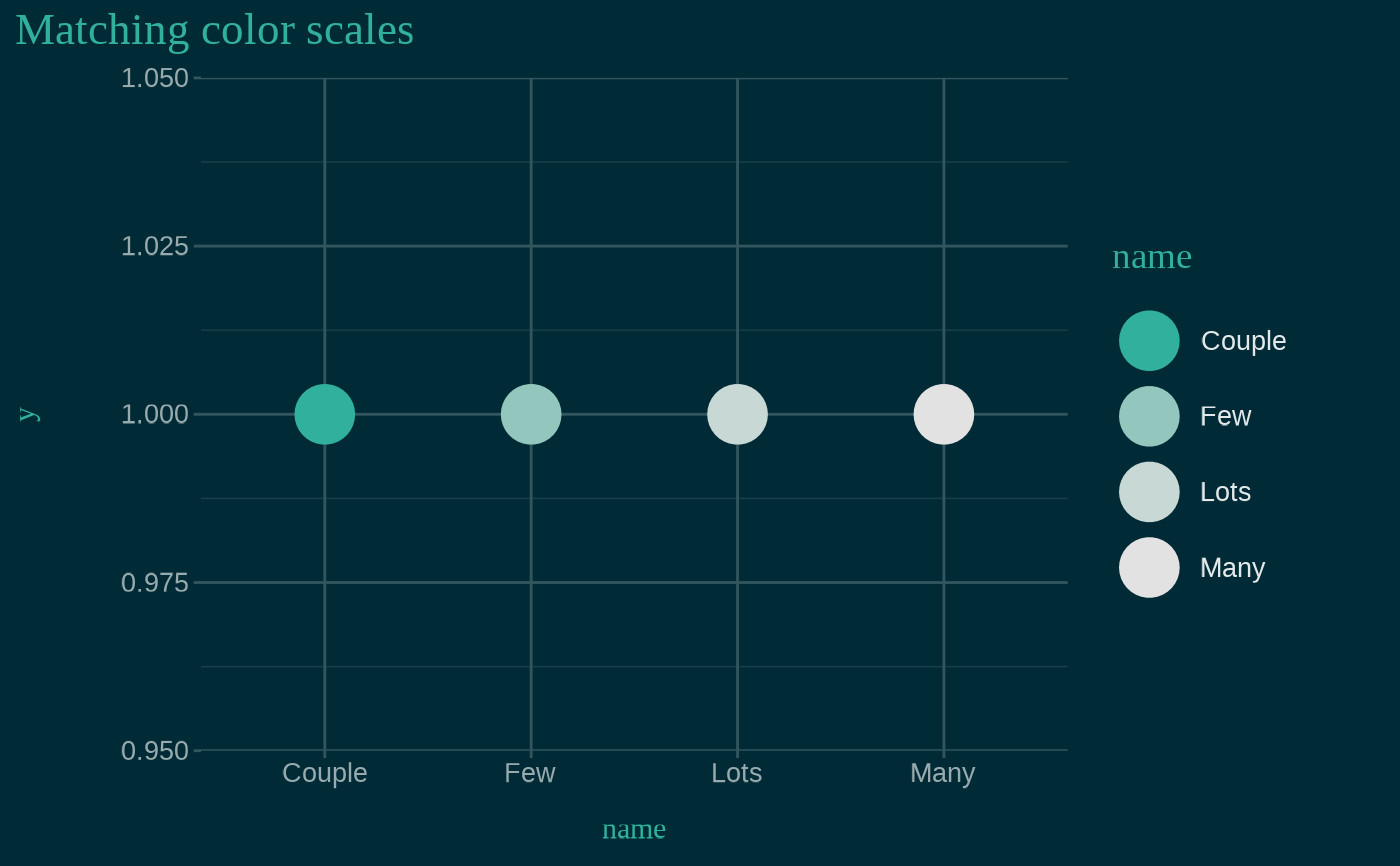Color and fill single-color scales for discrete and continuous values, created using the primary accent color of the xaringanthemer styles. See vignette("ggplot2-themes") for more information and examples of xaringanthemer's ggplot2-related functions.

Usage

scale_xaringan_discrete(
aes_type = c("color", "colour", "fill"),
...,
color = NULL,
direction = 1,
inverse = FALSE
)

scale_xaringan_fill_discrete(..., color = NULL, direction = 1, inverse = FALSE)

scale_xaringan_color_discrete(
...,
color = NULL,
direction = 1,
inverse = FALSE
)

scale_xaringan_colour_discrete(
...,
color = NULL,
direction = 1,
inverse = FALSE
)

scale_xaringan_continuous(
aes_type = c("color", "colour", "fill"),
...,
color = NULL,
begin = 0,
end = 1,
inverse = FALSE
)

scale_xaringan_fill_continuous(
...,
color = NULL,
begin = 0,
end = 1,
inverse = FALSE
)

scale_xaringan_color_continuous(
...,
color = NULL,
begin = 0,
end = 1,
inverse = FALSE
)

scale_xaringan_colour_continuous(
...,
color = NULL,
begin = 0,
end = 1,
inverse = FALSE
)

Arguments

aes_type

The type of aesthetic to which the scale is being applied. One of "color", "colour", or "fill".

...

Arguments passed on to either the colorspace scale functions — one of colorspace::scale_color_discrete_sequential(), colorspace::scale_color_continuous_sequential(), colorspace::scale_fill_discrete_sequential(), or colorspace::scale_fill_continuous_sequential() — or to ggplot2::continuous_scale or ggplot2::discrete_scale.

color

A color value, in hex, to override the default color. Otherwise, the primary color of the resulting scale is chosen from the xaringanthemer slide styles.

direction

Direction of the discrete scale. Use values less than 0 to reverse the direction, e.g. direction = -1.

inverse

If color is not supplied and inverse = TRUE, a primary color is chosen to work well with the inverse slide styles, namely the value of inverse_header_color

begin

Number in the range of [0, 1] indicating to which point in the color scale the smallest data value should be mapped.

end

Number in the range of [0, 1] indicating to which point in the color scale the largest data value should be mapped.

Examples

# Requires ggplot2
has_ggplot2 <- requireNamespace("ggplot2", quietly = TRUE)

if (has_ggplot2) {

# Saving the theme to a temp file because this is an example
path_to_css_file <- tempfile(fileext = ".css")

# Create the xaringan theme: dark blue background with teal green accents

primary_color = "#002b36",
secondary_color = "#31b09e",
# Using basic fonts for this example, but the plot theme will
text_font_family = "sans",
outfile = path_to_css_file
)

# Here's some very basic example data
ex <- data.frame(
name = c("Couple", "Few", "Lots", "Many"),
n = c(2, 3, 5, 7)
)

# Fill color scales demo
ggplot(ex) +
aes(name, n, fill = n) +
geom_col() +
ggtitle("Matching fill scales") +
# themed to match the slides: dark blue background with teal text

# Fill color matches teal text
scale_xaringan_fill_continuous()

# Color scales demo
ggplot(ex) +
aes(name, y = 1, color = name) +
geom_point(size = 10) +
ggtitle("Matching color scales") +
# themed to match the slides: dark blue background with teal text

# Fill color matches teal text
scale_xaringan_color_discrete(direction = -1)
}